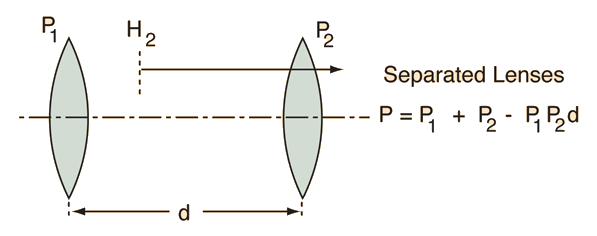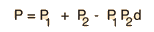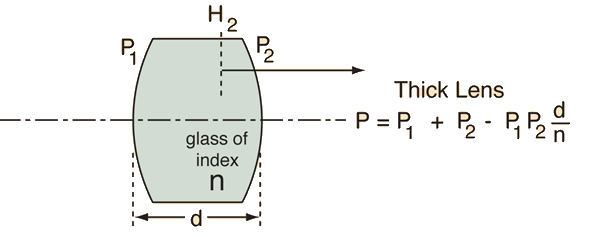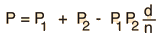# Gullstrand's Equation Calculations

Gullstrand's equation can be used to calculate the effective focal length of a thick lens or two separated lenses with respect to the second principal plane. It is often useful to calculate the front and back vertex powers or focal lengths.For lenses with powers P1 = diopters and P2= diopters
 with separation d = cm,
 the equivalent power given by Gullstrand's equation is= diopters
 and the focal length is f = cm.
 The front vertex focal length is cm
 and the back vertex focal length is cm.
 Ray diagram for two thin lenses

By putting in the index of refraction of the glass, Gullstrand's equation can be applied to a thick lens. The powers in the equation are then the surface powers.For a thick lens with surface powers P1 = and P2 = diopters
 with separation d = cm,
 and index of refraction n = ,
 the equivalent power given by Gullstrand's equation is= diopters
 and the focal length is f = cm.
 The front vertex focal length is cm
 and the back vertex focal length is cm.

Since Gullstrand powers and focal lengths are measured with respect to a hypothetical plane, it is often more useful to deal with front and back vertex focal lengths.

Index

Lens concepts

Thick lens concepts

 HyperPhysics***** Light and Vision R Nave
Go Back